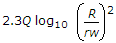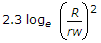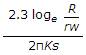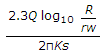# Civil Engineering - Water Supply Engineering

### Exercise :: Water Supply Engineering - Section 1

31.

Asbestos pipes are

 A. light in weight and easy to transport B. highly resistant to corrosion C. high flexible to accommodate deflection upto 12° D. very much smooth and hydraulically efficient E. all the above.

Explanation:

No answer description available for this question. Let us discuss.

32.

The maximum depth of sedimentation tanks is limited to

 A. 2 m B. 3 m C. 4 m D. 5 m E. 6 m.

Explanation:

No answer description available for this question. Let us discuss.

33.

By boiling water, hardness can be removed if it is due to

 A. calcium sulphate B. magnesium sulphate C. calcium nitrate D. calcium bicarbonate E. none of these.

Explanation:

No answer description available for this question. Let us discuss.

34.

Q is the discharge from an unconfined tube well with depression head s through its pipe of radius rw. If the radius of influence is R, the length of the required strainer, is

 A.B.C.D.Explanation:

No answer description available for this question. Let us discuss.

35.

For determining the velocity of flow of underground water, the most commonly used non-empirical formula is

 A. Darcy's formula B. Slichter's formula C. Hazen's formula D. Lacy's formula.